# CBSEtips.in

## Sunday, 28 February 2021

### CBSE Class 6 Maths - MCQ and Online Tests - Unit 13 - Symmetry

#### CBSE Class 6 Maths – MCQ and Online Tests – Unit 13 – Symmetry

Every year CBSE students attend Annual Assessment exams for 6,7,8,9,11th standards. These exams are very competitive to all the students. So our website provides online tests for all the 6,7,8,9,11th standards’ subjects. These tests are also very effective and useful for those who preparing for any competitive exams like Olympiad etc. It can boost their preparation level and confidence level by attempting these chapter wise online tests.

These online tests are based on latest CBSE syllabus. While attempting these, our students can identify their weak lessons and continuously practice those lessons for attaining high marks. It also helps to revise the NCERT textbooks thoroughly.#### CBSE Class 6 Maths – MCQ and Online Tests – Unit 13 – Symmetry

Question 1.
Which of the following letters has horizontal line of symmetry?
(a) C
(b) A
(c) J
(d) L.

Question 2.
How many lines of symmetry does the figure have ?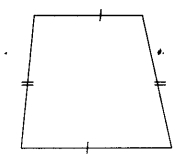(a) 1
(b) 2
(c) 3
(d) no line of symmetry

Question 3.
Which of the following letters has horizontal line of symmetry?
(a) Z
(b) V
(c) U
(d) E.

Question 4.
How many lines of symmetry does the figure have?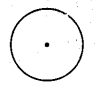(a) 1
(b) 2
(c) 3
(d) Countless.

Question 5.
Which of the following letters has vertical line of symmetry?
(a) R
(b) C
(c) B
(d) T.

Question 6.
How many lines of symmetry does the figure have?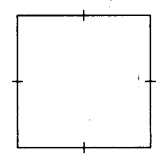(a) 1
(b) 2
(c) 3
(d) 4

Question 7.
How many lines of symmetry does the figure have ?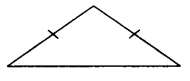(a) 1
(b) 2
(c) 3
(d) 4

Question 8.
How many lines of symmetry does the figure have?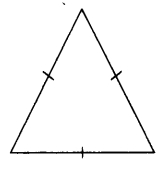(a) 1
(b) 2
(c) 3
(d) 4

Question 9.
How many lines of symmetry does the figure have?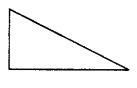(a) 0
(b) 1
(c) 2
(d) countless

Question 10.
How many lines of symmetry does the figure have?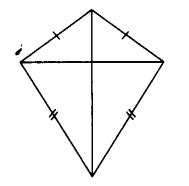(a) 1
(b) 2
(c) 3
(d) 4

Question 11.
How many lines of symmetry does the figure have?(a) 1
(b) 2
(c) 3
(d) 4

Question 12.
How many lines of symmetry does the figure have?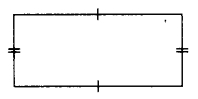(a) 1
(b) 2
(c) 3
(d) 4.

Question 13.
How many lines of symmetry does a regular hexagon have?
(a) 1
(b) 3
(c) 4
(d) 6

Question 14.
Which of the following letters has horizontal line of symmetry?
(a) S
(b) W
(c) D
(d) Y.

Question 15.
Which of the following letters has vertical line of symmetry?
(a) N
(b) K
(c) B
(d) M.